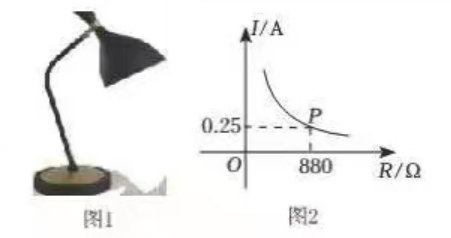$\text{A.}$ 当 $R < 0.25$ 时, $I < 880$ $\text{B.}$ $I$ 与 $R$ 的函数关系式是 $I=\frac{200}{\mathrm{R}}(R>0)$ $\text{C.}$ 当 $R>1000$ 时, $I>0.22$ $\text{D.}$ 当 $880 < R < 1000$ 时， $I$ 的取值范因是 $0.22 < I < 0.25$